• Posts tagged "熵"

# Blog Archives

## 信息熵的时间度量模型muti

R的极客理想系列文章，涵盖了R的思想，使用，工具，创新等的一系列要点，以我个人的学习和体验去诠释R的强大。

R语言作为统计学一门语言，一直在小众领域闪耀着光芒。直到大数据的爆发，R语言变成了一门炙手可热的数据分析的利器。随着越来越多的工程背景的人的加入，R语言的社区在迅速扩大成长。现在已不仅仅是统计领域，教育，银行，电商，互联网….都在使用R语言。

• 张丹(Conan), 程序员/Quant: Java,R,Nodejs
• blog: http://blog.fens.me
• email: bsspirit@gmail.com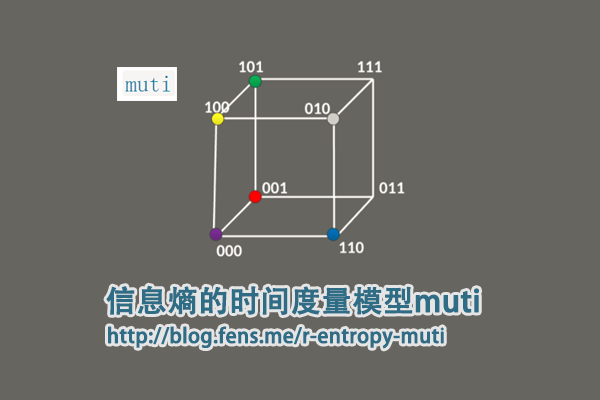1. muti包介绍
2. muti包函数定义
3. muti包使用
4. 实战案例

## 1. muti包介绍

muti包是用于计算两个离散随机变量之间的互信息(MI)的R包。 muti包设计目标，是考虑时间序列分析的情况下开发的，但没有任何方法可以将方法与时间索引本身联系起来。

muti项目的主页：https://mdscheuerell.github.io/muti/

muti包中用来计算互信息的计算过程，包括以下几步：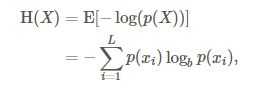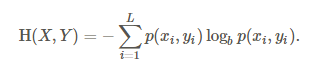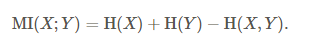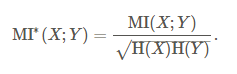• Win10 64bit
• R: 3.4.2 x86_64-w64-mingw32/x64 b4bit

muti目前还没有发布到CRAN上面，需要通过devtools包从github上进行安装。安装操作如下。

``````
> if(!require("devtools")) {
>  install.packages("devtools")
>  library("devtools")
> }
> devtools::install_github("mdscheuerell/muti")
``````

## 2. muti包函数定义

muti包，有很强的专业性，没有太多的函数定义，函数都是为了实现模型的算法。

• muti()，主函数，用于计算两个向量的互信息并画图输出
• mutual_info()，计算互信息
• newZ()，基于原始数据的重新采样创建新矢量。
• symbolize()，将数字向量转换为符号向量。
• transM()，估计用于计算互信息上的临界阈值的转换矩阵。
• plotMI()，画图输出

2.1 muti()函数：

``````
muti(x, y, sym = TRUE, n_bins = NULL, normal = FALSE, lags = seq(-4, 4), mc = 100, alpha = 0.05)
``````

• x, 向量
• y, 向量
• sym，是否转换成符号向量，默认TRUE,
• n_bins, 离散化方法，当sym=FALSE时， n_bins = ceiling（2 * length(x)^(1/3))
• normal, 是否标准化，默认FALSE
• lags, 滞后周期，默认为seq(-4, 4)
• mc, 用于估计互信息的临界阈值的蒙特卡洛数量的模拟，默认100,
• alpha, 用于定义临界阈值的取值点0.05

2.2 mutual_info()函数：

``````
# 源函数
mutual_info <- function(su,normal) {
## function to calculate mutual information between 2 time series
## need to remove obs with NA's b/c they don't contribute to info
su <- su[!apply(apply(su,1,is.na),2,any),]
## get number of bins
bb <- max(su)
## get ts length
TT <- dim(su)
## init entropies
H_s <- H_u <- H_su <- 0
for(i in 1:bb) {
## calculate entropy for 1st series
p_s <- sum(su[,1] == i)/TT
if(p_s != 0) { H_s <- H_s - p_s*log(p_s, 2) }
## calculate entropy for 2nd series
p_u <- sum(su[,2] == i)/TT
if(p_u != 0) { H_u <- H_u - p_u*log(p_u, 2) }
for(j in 1:bb) {
## calculate joint entropy
p_su <- sum(su[,1]==i & su[,2]==j)/TT
if(p_su != 0) { H_su <- H_su - p_su*log(p_su, 2) }
} ## end j loop
} ## end i loop
## calc mutual info
MI <- H_s + H_u - H_su
if(!normal) { return(MI) } else { return(MI/sqrt(H_s*H_u)) }
}

# 修改后的函数
library(philentropy)
mutual_info <- function(su,normal){
n<-nrow(su)
px<-table(su[,1])/n
py<-table(su[,2])/n

hX<-H(px)
hY<-H(py)

tf<-table(su[,1],su[,2])
pXY<-prop.table(tf)
hXY<-JE(pXY)

MI<-hX+hY-hXY
if(normal){
return(MI/sqrt(hX * hY))
}else{
return(MI)
}
}
``````

2.3 symbolize()函数：

``````
# 源函数
symbolize <- function(xy) {
## of interest and converts them from numeric to symbolic.
## check input for errors
if(dim(xy) != 2) {
stop("xy must be an [n x 2] matrix \n\n", call.=FALSE)
}
## get ts length
TT <- dim(xy)
## init su matrix
su <- matrix(NA, TT, 2)
## convert to symbols
## loop over 2 vars
for(i in 1:2) {
for(t in 2:(TT-1)) {
## if xy NA, also assign NA to su
if(any(is.na(xy[(t-1):(t+1),i]))) { su[t,i] <- NA }
## else get correct symbol
else {
if(xy[t,i] == xy[t-1,i] & xy[t,i] == xy[t+1,i]) {
## same
su[t,i] <- 3
}
if(xy[t,i] > xy[t-1,i]) {
## peak
if(xy[t,i] > xy[t+1,i]) { su[t,i] <- 5 }
## increase
else { su[t,i] <- 4 }
}
if(xy[t,i] < xy[t-1,i]) {
## trough
if(xy[t,i] < xy[t+1,i]) { su[t,i] <- 1 }
## decrease
else { su[t,i] <- 2 }
}
} ## end else
} ## end t loop
} ## end i loop
## return su matrix
return(su)
}

# 优化后的函数
symbolize<-function(xy){
if(ncol(xy)!=2) {
stop("xy must be an [n x 2] matrix \n\n", call. = FALSE)
}

idx<-2:(nrow(xy)-1)
apply(xy,2,function(row){
na<-which(is.na(row[idx]+row[idx-1]+row[idx+1]))
n3<-which(row[idx]==row[idx-1] & row[idx]==row[idx+1])
n5<-which(row[idx]>row[idx-1] & row[idx]>row[idx+1])
n4<-which(row[idx]>row[idx-1] & row[idx]<=row[idx+1])
n1<-which(row[idx]<row[idx-1] & row[idx]<row[idx+1])
n2<-which(row[idx]<row[idx-1] & row[idx]>=row[idx+1])

line<-rep(0,length(idx))
line[na]<-NA
line[n1]<-1
line[n2]<-2
line[n3]<-3
line[n4]<-4
line[n5]<-5
c(NA,line,NA)
})
}
``````

2.4 transM()函数：

``````
# 源函数
transM <- function(x,n_bins) {
## helper function for estimating transition matrix used in
## creating resampled ts for the CI on mutual info
## replace NA with runif()
x[is.na(x)] <- runif(length(x[is.na(x)]),min(x,na.rm=TRUE),max(x,na.rm=TRUE))
## length of ts
tt <- length(x)
## get bins via slightly extended range
bins <- seq(min(x)-0.001,max(x),length.out=n_bins+1)
## discretize ts
hin <- vector("numeric",tt)
for(b in 1:n_bins) {
hin[x > bins[b] & x <= bins[b+1]] <- b
}
## matrix of raw-x & discrete-x
xn <- cbind(x,hin)
## transition matrix from bin-i to bin-j
MM <- matrix(0,n_bins,n_bins)
for(i in 1:n_bins) {
for(j in 1:n_bins) {
MM[i,j] <- sum(hin[-tt]==i & hin[-1]==j)
}
if(sum(MM[i,])>0) { MM[i,] <- MM[i,]/sum(MM[i,]) }
else { MM[i,] <- 1/n_bins }
}
return(list(xn=xn,MM=MM))
} ## end function

# 优化后的函数
transM <- function(x,n_bins){
x[is.na(x)] <- runif(length(x[is.na(x)]), min(x, na.rm = TRUE),max(x, na.rm = TRUE))
n<-length(x)
xn<-cbind(x,hin=cut(x,7))

hin<-xn[,2]
a<-data.frame(id=1:(n-1),a=hin[1:(n-1)])
b<-data.frame(id=1:(n-1),b=hin[2:n])
ab<-merge(a,b,by="id",all=TRUE)
ab\$v<-1
abm<-aggregate(v~a+b,data=ab,sum)

MM <- matrix(0, n_bins, n_bins)
apply(abm,1,function(row){
MM[row,row]<<-row
invisible()
})

MN<-apply(MM,1,function(row){
s<-sum(row)
if(s>0){
row/s
}else{
rep(1/n_bins,n_bins)
}
})
MN<-t(MN)
return(list(xn = xn, MM = MN))
}
``````

## 3. muti包使用

3.1 数据的输入和输出
muti()函数，是muti包的主函数，调用muti()就完成了这个包的全部功能。

• 输入，要求是2个数字型向量。
• 输出，包括了2个部分，1.数据计算结果，2.可视化的结果。

``````> set.seed(123)
> TT <- 30

# 创建两个数字型向量
> x1 <- rnorm(TT)
> y1 <- x1 + rnorm(TT)

# 计算互信息
> muti(x1, y1)
lag MI_xy MI_tv
1  -4 0.238 0.595
2  -3 0.473 0.532
3  -2 0.420 0.577
4  -1 0.545 0.543
5   0 0.712 0.584
6   1 0.393 0.602
7   2 0.096 0.546
8   3 0.206 0.573
9   4 0.403 0.625
``````

• lag: 滞后期，当lag=-1，length(x)=length(y)=26时，x取值[1:25],y取值[2:26]；当lag=1，length(x)=length(y)=26时，x取值[2:26],y取值[1:25]；
• MI_xy: 互信息
• MI_tv: 相应显着性阈值，用蒙特卡洛方法生成互信息，按从大到小排序后取1-alpha位置的值。
• 上图，原始数据的x,y两个变量
• 中图，符号转型后的x,y两个变量，把原始数据离散化为5个值，差异化更大
• 下图，滞后期的互信息（实线）和相应显着性阈值（虚线），同数据计算结果的输出。

3.2 离散化处理

muti包提供了2种离散化。

• 符号化： 把连续型的数据，映射到5个值的上，分别对应peak(峰值), increasing(增加), same(没变)，decreasing(减少)，trough(低谷)。比如，向量c(1.1,2.1,3.3,1.2,3.1)，不计算第一个值和最后一个值，第二个值2.1>第一个值1.1，第二个值2.1<第三个值3.3时，第二个值为增加。第2，3，4值，分别为c(“增加”，“峰值”，“低谷”)。
• 分箱法： 等宽分箱，如果没有指定箱数，则根据莱斯规则计算，其中n = ceiling(2*length(x)^(1/3))。

## 4. 实战案例

``````// 省略部分代码
date X601398 X000001
1  2014-10-21    2.92 2339.66
2  2014-10-22    2.91 2326.55
3  2014-10-23    2.92 2302.42
4  2014-10-24    2.92 2302.28
5  2014-10-27    2.90 2290.44
6  2014-10-28    2.92 2337.87
7  2014-10-29    2.94 2373.03
8  2014-10-30    2.98 2391.08
9  2014-10-31    3.04 2420.18
10 2014-11-03    3.01 2430.03
11 2014-11-04    3.02 2430.68
12 2014-11-05    2.97 2419.25
13 2014-11-06    2.97 2425.86
14 2014-11-07    2.97 2418.17
15 2014-11-10    3.05 2473.67
16 2014-11-11    3.16 2469.67
17 2014-11-12    3.12 2494.48
18 2014-11-13    3.12 2485.61
19 2014-11-14    3.14 2478.82
20 2014-11-17    3.11 2474.01
``````

3列数据，第一列是时间列，第二列是X601398人收盘价，第三列是X000001收盘价。从价格上看，2个数据集并不在同一个度量内，我们转化度量单位为每日收益率。

``````> head(ret,20)
X601398       X000001
2014-10-21           NA            NA
2014-10-22 -0.003424658 -5.603378e-03
2014-10-23  0.003436426 -1.037158e-02
2014-10-24  0.000000000 -6.080559e-05
2014-10-27 -0.006849315 -5.142728e-03
2014-10-28  0.006896552  2.070781e-02
2014-10-29  0.006849315  1.503933e-02
2014-10-30  0.013605442  7.606309e-03
2014-10-31  0.020134228  1.217023e-02
2014-11-03 -0.009868421  4.069945e-03
2014-11-04  0.003322259  2.674864e-04
2014-11-05 -0.016556291 -4.702388e-03
2014-11-06  0.000000000  2.732252e-03
2014-11-07  0.000000000 -3.170010e-03
2014-11-10  0.026936027  2.295124e-02
2014-11-11  0.036065574 -1.617031e-03
2014-11-12 -0.012658228  1.004588e-02
2014-11-13  0.000000000 -3.555851e-03
2014-11-14  0.006410256 -2.731724e-03
2014-11-17 -0.009554140 -1.940439e-03
``````

``````> head(rdf,20)
X601398       X000001
2014-10-22 -0.003424658 -0.0056033783
2014-10-23  0.000000000 -0.0159168426
2014-10-24  0.000000000 -0.0159766804
2014-10-27 -0.006849315 -0.0210372447
2014-10-28  0.000000000 -0.0007650684
2014-10-29  0.006849315  0.0142627561
2014-10-30  0.020547945  0.0219775523
2014-10-31  0.041095890  0.0344152569
2014-11-03  0.030821918  0.0386252703
2014-11-04  0.034246575  0.0389030885
2014-11-05  0.017123288  0.0340177633
2014-11-06  0.017123288  0.0368429601
2014-11-07  0.017123288  0.0335561577
2014-11-10  0.044520548  0.0572775531
2014-11-11  0.082191781  0.0555679030
2014-11-12  0.068493151  0.0661720079
2014-11-13  0.068493151  0.0623808588
2014-11-14  0.075342466  0.0594787277
2014-11-17  0.065068493  0.0574228734
2014-11-18  0.058219178  0.0498833164
``````

``````
> x<-rdf\$X601398
> y<-rdf\$X000001
> result<-muti(x,y)
> result
lag MI_xy MI_tv
1  -4 0.148 0.534
2  -3 0.158 0.464
3  -2 0.190 0.385
4  -1 0.351 0.425
5   0 0.646 0.398
6   1 0.528 0.340
7   2 0.262 0.461
8   3 0.207 0.437
9   4 0.260 0.518
``````
• 上图，为原始数据的x(上证综指)和y(工商银行)的累积收益率曲线。
• 中图，为符号转型后的x(上证综指)和y(工商银行)的累积收益率曲线。
• 下图，符号转型后的数据，滞后期从-4到4的9个的互信息（实线）和和相应显着性阈值（虚线）。

``````// 省略部分代码
> rsdf
lag MI_xy MI_tv       sd
1  -4 0.148 0.534 1.991886
2  -3 0.158 0.464 2.400721
3  -2 0.190 0.385 2.107189
4  -1 0.351 0.425 2.378768
5   0 0.646 0.398 1.477030
6   1 0.528 0.340 2.342482
7   2 0.262 0.461 1.997676
8   3 0.207 0.437 2.267211
9   4 0.260 0.518 2.116934
``````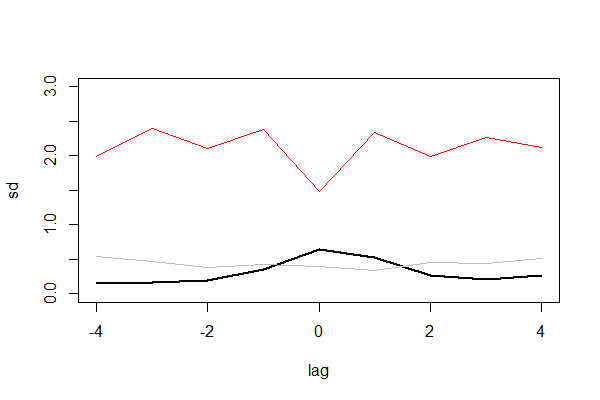## 用R语言实现信息度量

R的极客理想系列文章，涵盖了R的思想，使用，工具，创新等的一系列要点，以我个人的学习和体验去诠释R的强大。

R语言作为统计学一门语言，一直在小众领域闪耀着光芒。直到大数据的爆发，R语言变成了一门炙手可热的数据分析的利器。随着越来越多的工程背景的人的加入，R语言的社区在迅速扩大成长。现在已不仅仅是统计领域，教育，银行，电商，互联网….都在使用R语言。

• 张丹(Conan), 程序员/Quant: Java,R,Nodejs
• blog: http://blog.fens.me
• email: bsspirit@gmail.com

http://blog.fens.me/r-entropy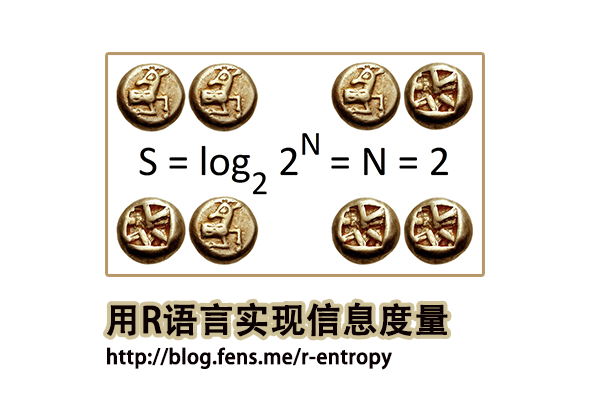1. 信息熵介绍
2. 关键概念
3. 信息度量函数
4. 应用举例

## 1.信息熵介绍

``````H(A) = -1*(0.2*log2(0.2)*5)
= 2.321928 bits``````

``````
H(B)=-1*(0.1*log2(0.1)+0.2*log2(0.2)+0.45*log2(0.45)+0.15*log2(0.15)+0.1*log2(0.1))
= 2.057717 bits
``````

## 2. 关键概念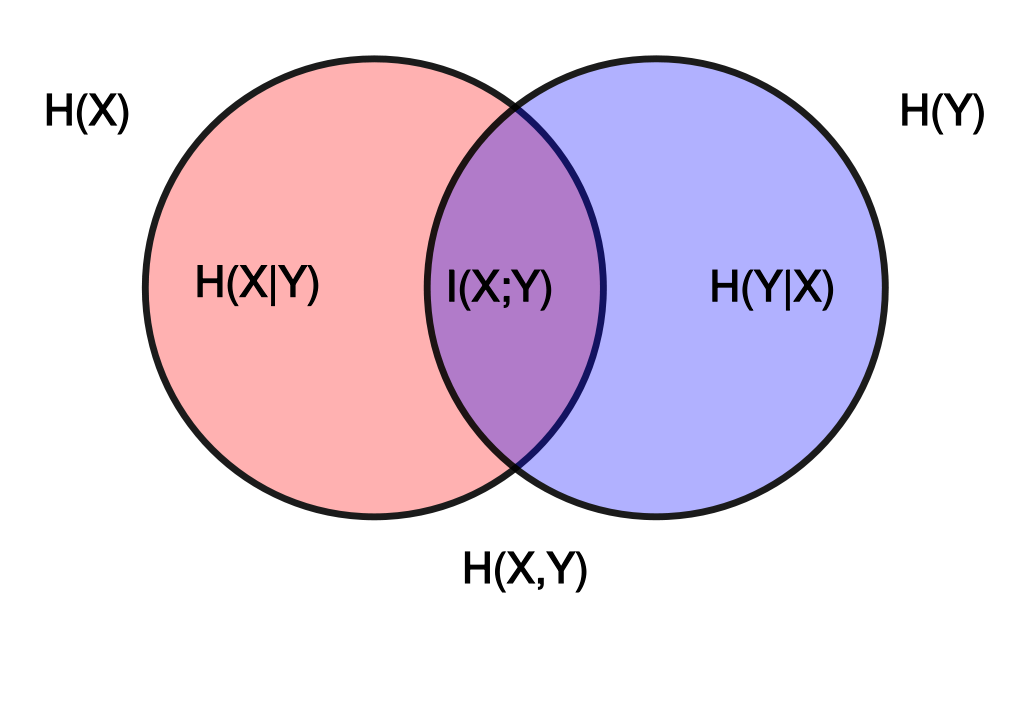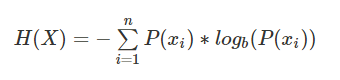H(x)的取值范围，0<=H(x)<=log(n), 其中n是随机变量x取值的种类数。需要注意的是，熵只依赖于随机变量的分布，与随机变量取值无关。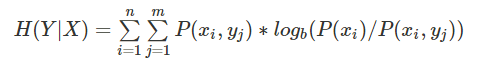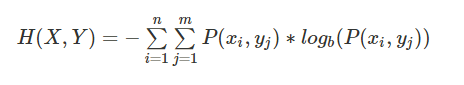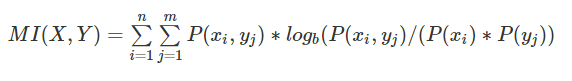KL散度（Kullback–Leibler Divergence, 相对熵）：随机变量x取值的两个概率分布p和q，用来衡量这2个分布的差异，通常用p表示真实分布，用q表示预测分布。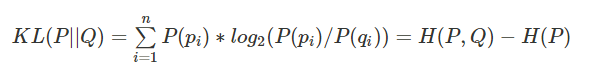n为事件的所有可能性，如果两个分布完全相同,那么它们的相关熵为0。如果相对熵KL越大，说明它们之间的差异越大，反之相对熵KL越小，说明它们之间的差异越小。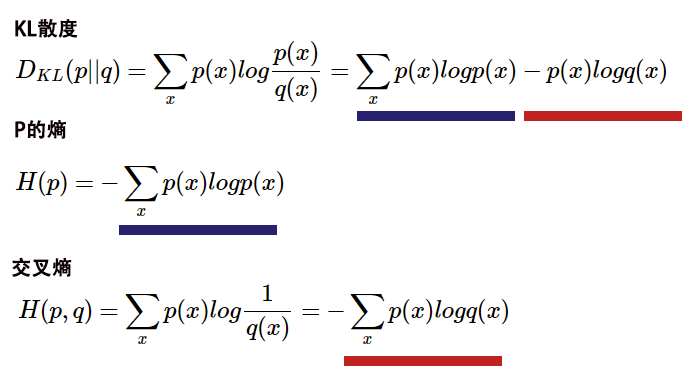## 3. 信息度量函数

philentropy包的函数，主要分为3种类别的函数，第一类是距离测量的函数，第二类是相关性分析，第三类是信息度量函数，本文重点介绍这些信息度量的函数。有关于距离测量函数和相关性分析函数，请参考文章R语言实现46种距离算法

• H(): 香农熵, Shannon’s Entropy H(X)
• JE() : 联合熵, Joint-Entropy H(X,Y)
• CE() : 条件熵, Conditional-Entropy H(X|Y)
• MI() : 互信息, Shannon’s Mutual Information I(X,Y)
• KL() : KL散度, Kullback–Leibler Divergence
• JSD() : JS散度，Jensen-Shannon Divergence
• gJSD() : 通用JS散度，Generalized Jensen-Shannon Divergence

• Win10 64bit
• R: 3.4.2 x86_64-w64-mingw32

3.1 H()香农熵
H()函数，可用于快速计算任何给定概率向量的香农熵。

H()函数定义：

``````H (x, unit = "log2")
``````

• x, 概率向量
• unit，对数化的单位，默认为log2

``````
# 创建数据x
> x<-1:10;x
  1  2  3  4  5  6  7  8  9 10
> px<-x/sum(x);x1
 0.01818182 0.03636364 0.05454545 0.07272727
 0.09090909 0.10909091 0.12727273 0.14545455
 0.16363636 0.18181818

# 计算香农熵
> H(px)
 3.103643
``````

``````
# 创建数据x
> x<-1:10
#计算x的概率密度px
> px<-x/sum(x)

# 根据公式计算香农熵
> -1*sum(px*log2(px))
 3.103643
``````

3.2 CE()条件熵

CE()函数，基于给定的联合概率向量P(X,Y)和概率向量P(Y)，根据公式 H(X|Y)= H(X,Y)-H(Y)计算香农的条件熵。

``CE(xy, y, unit = "log2")``

• xy, 联合概率向量
• y, 概率向量，必须是随机变量y的概率分布
• unit，对数化的单位，默认为log2

``````
> x3<- 1:10/sum(1:10)
> y3<- 30:40/sum(30:40)

# 计算条件熵
> CE(x3, y3)
 -0.3498852
``````

3.3 JE()联合熵

JE()函数，基于给定的联合概率向量P(X,Y)计算香农的联合熵H(X,Y)。

JE()函数定义：

``````JE (x, unit = "log2")
``````

• x, 联合概率向量
• unit，对数化的单位，默认为log2

``````# 创建数据x
> x2 <- 1:100/sum(1:100)

# 联合熵
> JE(x2)
 6.372236
``````

3.4 MI()互信息
MI()函数，根据给定联合概率向量P(X,Y)、概率向量P(X)和概率向量P(X)，按公式I(X,Y)= H(X)+ H(Y)-H(X,Y)计算。

``MI(x, y, xy, unit = "log2")``

• x, 概率向量
• x, 概率向量
• xy, 联合概率向量
• unit，对数化的单位，默认为log2

``````
# 创建数据集
> x3 <- 1:10/sum(1:10)
> y3<- 20:29/sum(20:29)
> xy3 <- 1:10/sum(1:10)

# 计算互信息
> MI(x3, y3, xy3)
 3.311973
``````

3.5 KL()散度
KL()函数，计算两个概率分布P和Q的Kullback-Leibler散度。

``KL(x, test.na = TRUE, unit = "log2", est.prob = NULL)``

• x, 概率向量或数据框
• test.na, 是否检查NA值
• unit，对数化的单位，默认为log2
• est.prob, 用计数向量估计概率的方法，默认值NULL。

``````
# 创建数据集
> df4 <- rbind(x3,y3);df4
[,1]       [,2]       [,3]       [,4]       [,5]      [,6]      [,7]      [,8]      [,9]
x3 0.01818182 0.03636364 0.05454545 0.07272727 0.09090909 0.1090909 0.1272727 0.1454545 0.1636364
y3 0.08163265 0.08571429 0.08979592 0.09387755 0.09795918 0.1020408 0.1061224 0.1102041 0.1142857
[,10]
x3 0.1818182
y3 0.1183673

# 计算KL散度
> KL(df4, unit = "log2") # Default
kullback-leibler
0.1392629
> KL(df4, unit = "log10")
kullback-leibler
0.0419223
> KL(df4, unit = "log")
kullback-leibler
0.09652967
``````

3.5 JSD()散度

JSD()函数，基于具有相等权重的Jensen-Shannon散度，计算距离矩阵或距离值。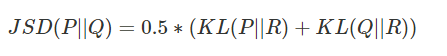``JSD(x, test.na = TRUE, unit = "log2", est.prob = NULL)``

• x, 概率向量或数据框
• test.na, 是否检查NA值
• unit, 对数化的单位，默认为log2
• est.prob, 用计数向量估计概率的方法，默认值NULL。
``````
# 创建数据
> x5 <- 1:10
> y5 <- 20:29
> df5 <- rbind(x5,y5)

# 计算JSD
> JSD(df5,unit='log2')
jensen-shannon
50.11323
> JSD(df5,unit='log')
jensen-shannon
34.73585
> JSD(df5,unit='log10')
jensen-shannon
15.08559

# 计算JSD，满足est.prob
> JSD(df5, est.prob = "empirical")
jensen-shannon
0.03792749
``````

3.6 gJSD()散度

gJSD()函数，计算概率矩阵的广义Jensen-Shannon散度。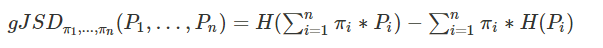``gJSD(x, unit = "log2", weights = NULL)``

• x, 概率矩阵
• unit, 对数化的单位，默认为log2
• weights, 指定x中每个值的权重，默认值NULL。
``````
# 创建数据
> Prob <- rbind(1:10/sum(1:10), 20:29/sum(20:29), 30:39/sum(30:39))

# 计算gJSD
> gJSD(Prob)
 0.023325
``````

## 4. 应用举例

``````
X,Y
Tourism,Y
Food,N
IT,Y
Tourism,N
Tourism,N
IT,Y
Food,N
Tourism,Y
``````

 X Y N p(X) Tourism 2/8=0.25 2/8=0.25 0.25+0.25=0.5 Food 0/8=0 2/8=0.25 0+0.25=0.25 IT 2/8=0.25 0/8=0 0.25+0=0.25 p(Y) 0.25+0+0.25=0.5 0.25+0.25+0=0.5

``````
# 分别计算每种情况的概率
p(X=Tourism) = 2/8 + 2/8 = 0.5
p(X=Food) = 2/8 + 0/8 = 0.25
p(X=IT) = 0/8 + 2/8 = 0.25
p(Y=Y) = 4/8 = 0.5
p(Y=N) = 4/8 = 0.5

# 计算H(X)
H(X) = -∑p(xi)*log2(p(xi))
= -p(X=Tourism)*log2(p(X=Tourism) ) -p(X=Food)*log2(p(X=Food) ) -p(X=IT)*log2(p(X=IT) )
= -0.5*log(0.5) -0.25*log(0.25) - 0.25*log(0.25)
= 1.5

# 计算H(Y)
H(Y) = -∑p(yi)*log2(p(yi))
= -p(Y=Y)*log2(p(Y=Y)) -p(Y=N)*log2(p(Y=N))
= -0.5*log(0.5) -0.5*log(0.5)
= 1
``````

``````
H(Y|X=Tourism) = -p(Y|X=Tourism)*log(p(Y|X=Tourism)) - p(N|X=Tourism)*log(p(N|X=Tourism))
= -0.5*log(0.5) -0.5*log(0.5)
= 1

H(Y|X=Food) = -p(Y|X=Food)*log(p(Y|X=Food)) -p(N|X=Food)*log(p(N|X=Food))
= -0*log(0) -1*log(1)
= 0

H(Y|X=IT) = -p(Y|X=IT)*log(p(Y|X=IT)) -p(N|X=IT)*log(p(N|X=IT))
= -1*log(1) -0*log(0)
= 0
``````

``````
H(Y|X) = ∑p(xi)*H(Y|xi)
= p(X=Tourism)*H(Y|X=Tourism) + p(X=Food)*H(Y|X=Food) + p(X=IT)*H(Y|X=IT)
= 0.5*1 + 0.25*0 + 0.25*0
= 0.5
``````

``````
H(X,Y) = −∑p(x,y)log(p(x,y))
= H(X) + H(Y|X)
= 1.5 + 0.5
= 2
``````

``````
I(X;Y) = H(Y) - H(Y|X)  = 1 - 0.5 = 0.5
= H(X) + H(Y) - H(X,Y) = 1.5 + 1 - 2 = 0.5
``````

``````
# 创建数据集
> X<-c('Tourism','Food','IT','Tourism','Tourism','IT','Food','Tourism')
> Y<-c('Y','N','Y','N','N','Y','N','Y')
> df<-cbind(X,Y);df
X         Y
[1,] "Tourism" "Y"
[2,] "Food"    "N"
[3,] "IT"      "Y"
[4,] "Tourism" "N"
[5,] "Tourism" "N"
[6,] "IT"      "Y"
[7,] "Food"    "N"
[8,] "Tourism" "Y
``````

``````
> tf<-table(df[,1],df[,2]);tf

N Y
Food    2 0
IT      0 2
Tourism 2 2
``````

``````
> pX<-margin.table(tf,1)/margin.table(tf);pX
Tourism    Food      IT
0.50    0.25    0.25
> pY<-margin.table(tf,2)/margin.table(tf);pY
Y   N
0.5 0.5
> pXY<-prop.table(tf);pXY
Y    N
Tourism 0.25 0.25
Food    0.00 0.25
IT      0.25 0.00
``````

``````
> H(pX)
 1.5
> H(pY)
 1

# 条件熵
> CE(pX,pY)
 0.5

# 联合熵
> JE(pXY)
 2

# 互信息
> MI(pX,pY,pXY)
 0.5
``````

http://blog.fens.me/r-entropy